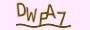# 7个JavaScript面试问题进行练习

268 0

## JavaScript面试问答

### forEach循环和.map（）函数之间有什么区别？

forEach循环为列表中的每个元素执行一个回调函数。一旦运行，它将不返回任何值。map语句在列表中的每个元素上调用一个函数，并返回具有该函数返回的值的转换数组。

### 如何检查数组是否为空？

`if (array_name === undefined || array_name.length === 0) {	// Code to run}`

### 函数中的默认参数是什么？

`function multiply_numbers(number_one = 0, number_two = 0) {	return number_one * number_two;}`

### 什么是箭头功能？

`const multiply_values = (value1, value2) => console.log(value1 * value2);`

### 如何从对象数组中过滤出值？

`var students = [ { name: “Sam”, grade: 6 }, { name: “Alix”, grade: 7 }];`

`var sixth_grade = students.filter(student => (student.grade === 6));`

`{ name: “Sam”, grade: 6 }`

### 什么是函数式编程？

JavaScript支持功能和面向对象的编程。函数式编程是一种编程范例，其中使用纯函数编写程序。

•